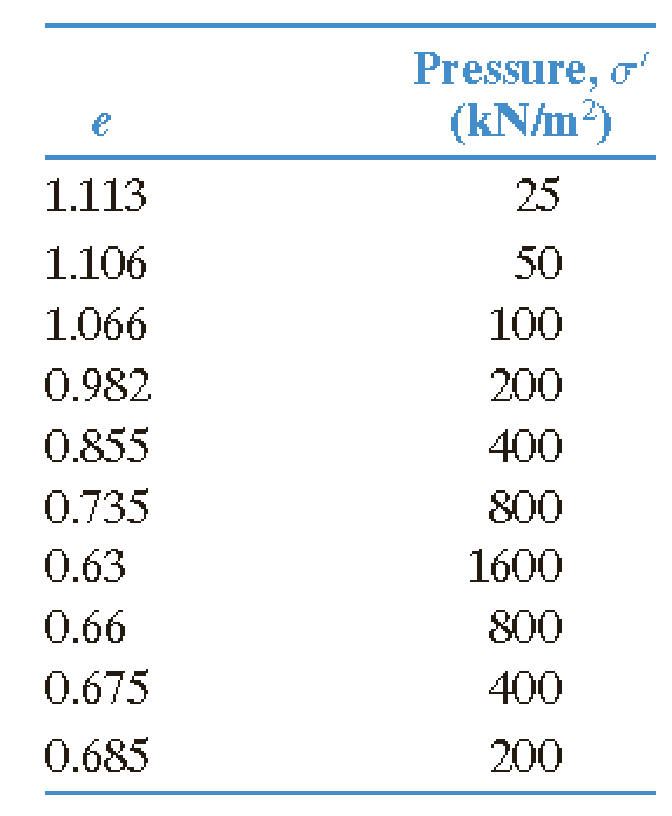Chapter 11, Problem 11.4PPrinciples of Geotechnical Enginee...

9th Edition
Braja M. Das + 1 other
ISBN: 9781305970939

Solutions

Chapter
SectionPrinciples of Geotechnical Enginee...

9th Edition
Braja M. Das + 1 other
ISBN: 9781305970939
Textbook Problem

The following are the results of a consolidation test on a sample of a clayey soil.a. Plot the e-log σ′ curve.b. Using Casagrande’s method, determine the preconsolidation pressure.c. Calculate the compression index, Cc and the ratio of Cs/Cc.

(a)

To determine

Plot the elogσ curve.

Explanation

Given information:

The results (pressure and void ratio) of consolidation test on a sample of a clayey soil are given in the Table.

Calculation:

Sketch the elogσ graph using the Table values

(b)

To determine

Calculate the preconsolidation pressure using Casagrande’s method.

(c)

To determine

Calculate the compression index CC

Calculate the ratio of swell index to compression index CSCC.

Still sussing out bartleby?

Check out a sample textbook solution.

See a sample solution

The Solution to Your Study Problems

Bartleby provides explanations to thousands of textbook problems written by our experts, many with advanced degrees!

Get Started

What are three benefits of precision grinding?

Precision Machining Technology (MindTap Course List)

Determine the largest angle for which the homogeneous block remains at rest.

International Edition---engineering Mechanics: Statics, 4th Edition

What is the difference between a detail report, a summary report, and an exception report?

Systems Analysis and Design (Shelly Cashman Series) (MindTap Course List)